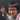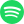# Discrete Calculus: Definition of Discrete Derivative

May 30, 2020 · 16:45 pm · Discrete CalculusMath

We have learned about the operator $\Delta$ being used for a change in a variable, probably in a science class. However, your teachers probably handwaved them as something that means “a change in”. But what does it really mean?

According to Wikipedia, this is known as a finite difference. The formal notation uses a subscript to show the size of the difference between arguments like so:

$\Delta_h f(x) = f(x+h) - f(x)$

There are alternative definitions since we can start and end anywhere. The above is known as a forward difference since we substract the current value $f(x)$ from the next value $f(x+h)$.

There is also the backward difference:

$\nabla_h f(x) = f(x) - f(x - h)$

And the central difference:

$\delta_h f(x) = f\left(x + \frac{h}{2}\right) - f\left(x - \frac{h}{2}\right)$

The Calculus Wiki suggests that we can omit the subscript if $h = 1$:

$\Delta f(x) = f(x + 1) - f(x)$

However, omitting the subscript is also used for other cases, e.g. in physics to denote the change from the initial state to the final state, so it’s probably a good idea to clarify. Note that we can define conventional derivatives using finite differences, and along the way also get an informal intuition about derivatives. We can start from the definition and go from there:

\begin{aligned} \frac{df}{dx} &= \lim_{h \to 0} \frac{f(x + h) - f(x)}{h} \\ &= \lim_{h \to 0} \frac{\Delta_h f(x)}{h} \\ \end{aligned}

Let $I(x)=x$, or if you’re feeling frisky, $I \colon x \mapsto x$. Then,

\begin{aligned} \frac{df}{dx} &= \lim_{h \to 0} \frac{\Delta_h f(x)}{h} \\ &= \lim_{h \to 0} \frac{\Delta_h f(x)}{h} \\ &= \lim_{h \to 0} \frac{\Delta_h f(x)}{0+h - 0} \\ &= \lim_{h \to 0} \frac{\Delta_h f(x)}{I(0 + h) - I(0)} \\ &= \lim_{h \to 0} \frac{\Delta_h f(x)}{\Delta_h I(0)} \\ \end{aligned}

We can establish a few things with the final equation, $\frac{df}{dx} = \lim_{h \to 0} \frac{\Delta_h f(x)}{\Delta_h I(0)}$. Firstly, since $\lim$ doesn’t distribute, we can’t directly define $df$ or $dx$ with $\Delta_h f$ or $\Delta_h x$. That is, the differential operator, $d$, inherently compares the change in rate of multiple functions. Therefore, it makes sense to talk about $\frac{df}{dx}$, but not $df$.1

Secondly, we can have an informal intuition about what $df$ and $dx$ really means. $df$ is associated with $\Delta_h f(x)$ and $dx$ is associated with $\Delta_h I(0) = h$ as $h \to 0$.

Lastly, we can define the “discrete derivative” as when $h=1$, leading to

\begin{aligned} \frac{\Delta_1 f(x)}{\Delta_1 I(0)} &= \frac{\Delta f(x)}{I(1) - I(0)} \\ &= \frac{\Delta f}{1 - 0} \\ &= \Delta f \\ \end{aligned}

Interestingly, for discrete derivatives, we don’t need a “denominator” in our differential expression.

1. Of course, you can always be working in a system that explicitly defines differentials.Streaming direct thought dumps from Yuto Nishida.
Connect with me on LinkedIn! I'm currently listening to: